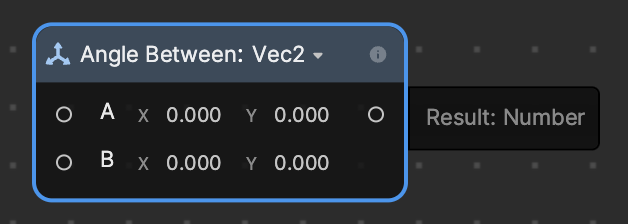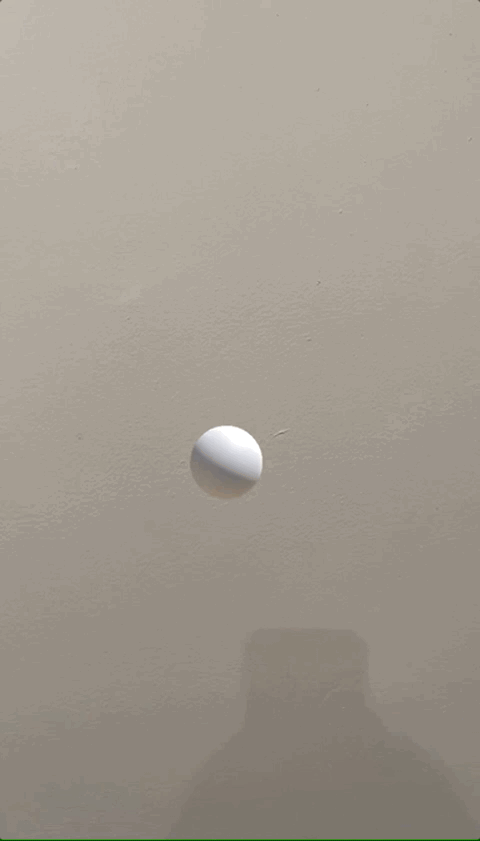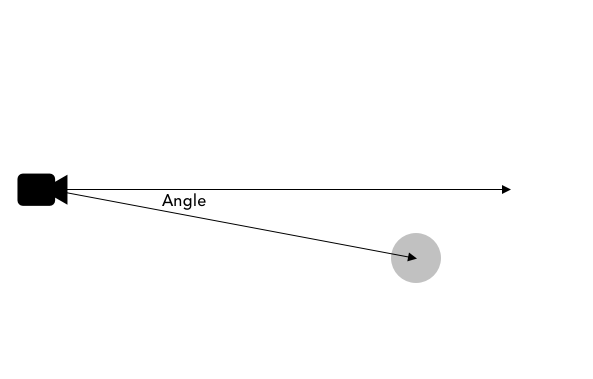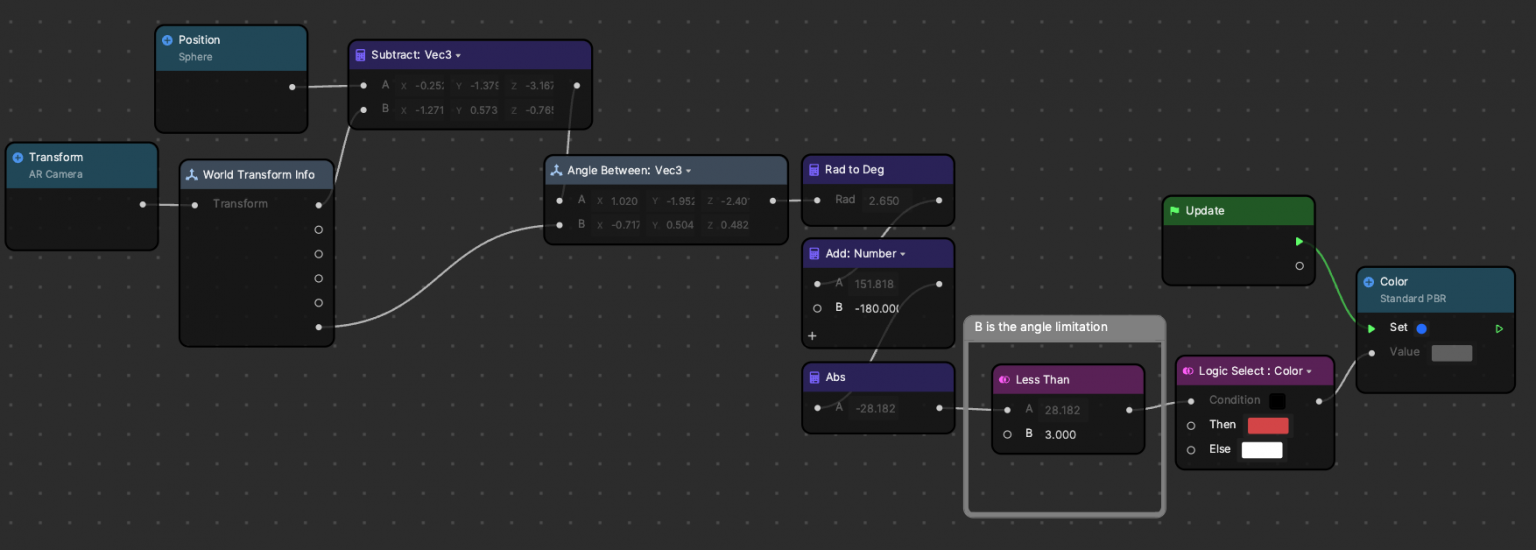Version: 3.5.0

# Angle BetweenUse Angle Between to calculate the angle between two vectors. Supported types: Vec2 and Vec3. Angle Between is in the Transform category.

## Input​

NameData TypeDescription
AVec2The first vector.
BVec2The second vector.

## Output​

NameData TypeDescription
ResultNumberThe acute angle between the two input vectors in radians.

## Example​↓ angle_between.zip

This example shows how to use Angle Between to detect if the ray from the camera is hitting the sphere within the 5 degrees. If it is, the sphere will turn red.